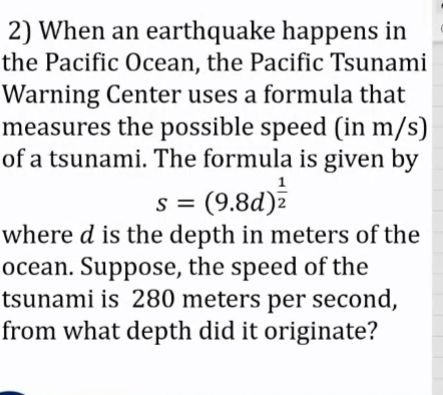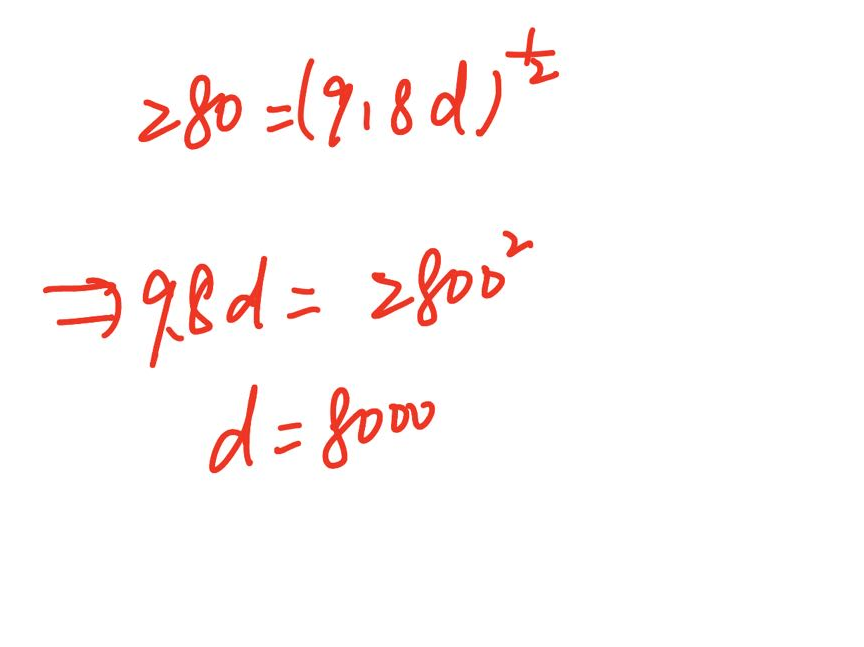### ¿Todavía tienes preguntas de matemáticas?

Pregunte a nuestros tutores expertos
Algebra
Pregunta2) When an earthquake happens in the Pacific Ocean, the Pacific Tsunami Warning Center uses a formula that measures the possible speed (in $$m / s$$ ) of a tsunami. The formula is given by

$$s = ( 9.8 d ) ^ { \frac { 1 } { 2 } }$$

where $$d$$ is the depth in meters of the ocean. Suppose, the speed of the tsunami is $$280$$ meters per second, from what depth did it originate?